Subsections

# Landau Theory

## Main Idea

From previous section we learned that:

1.
Free energy A is an analytical function of magnetization M
2.
At T>Tc it has one minimum, at T<Tc--two

Landau idea: let us use only these facts!

## Expansion

Let us expand free energy A in powers of M.

First, a symmetric system: H=0. Then M=0 is either a minimum (T>Tc) or a maximum (T<Tc) of the free energy. Due to symmetry only even powers are present: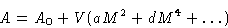Assumption:
We neglect all powers higher than 4.
At large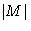free energy should be large--so d>0. We will see below that at a>0 there is one phase, at a<0--two. So a should change sign at T=Tc. We will expand it: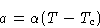With the same accuracy:Result: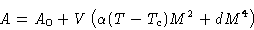(9)

## Phase Diagram

Differentiate (9):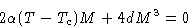Solutions:(10)
At T>Tc only the first solution is realized--one phase. At T<Tc we have two solutions--two phases: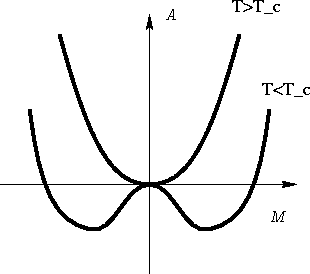Equation of binodal is (10):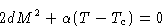(11)
This is parabola.

Equation of spinodal: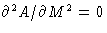or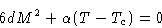(12)
This is another parabola, inside the binodal

Free energy: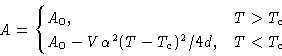(13)

## Entropy and Specific Heat

Entropy is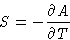Differentiating (13):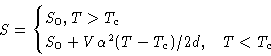Specific heat: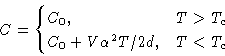There is a jump in specific heat near Tc: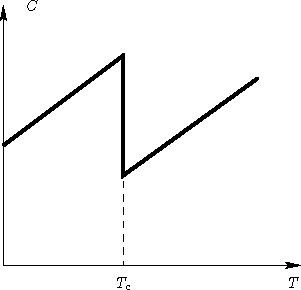## Susceptibility

Now we add a small external field H: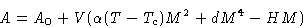(14)
and want to calculate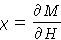Minimizing (14):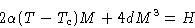(15)

Two cases:

1.
If T>Tc, then M(H=0)=0. For small H we neglect M3, and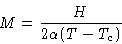Susceptibility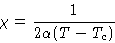This diverges at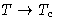.

2.
If T<Tc, then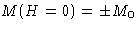with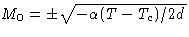. Let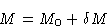Expanding (15), we obtain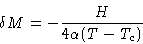and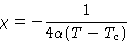This also diverges

We obtained a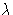-like curve: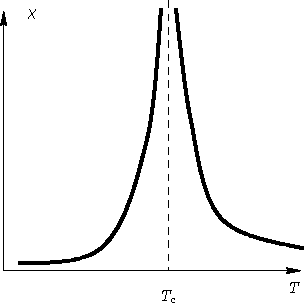Next: Quiz Up: Mean Field Approach Previous: Self-Consistent Field Theory

© 1997 Boris Veytsman and Michael Kotelyanskii
Tue Oct 14 22:58:59 EDT 1997